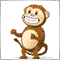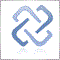# how to make library67

hi i have a indicator how to call my indicator function in to my EA?

Thank you.129167

Aleksey Pak:
thank you67

how do add this function in my EA.

any help & this is not my code i download from somewhere ???

//+------------------------------------------------------------------+
//+------------------------------------------------------------------+
#property version   "1.00"
#property description "Font, location and normalization can be defined in input parameters."
//---
//--- no graphical constructions
#property indicator_plots   0
//---
input color font_color=Red;
input int font_size=14;
input string font_face="Arial";
input ENUM_ANCHOR_POINT corner=ANCHOR_LEFT_UPPER;
input bool normalize=false; //If true then the spread is normalized to traditional pips
//---
double Poin;
int n_digits=0;
double divider=1;
//+------------------------------------------------------------------+
//| Custom indicator initialization function                         |
//+------------------------------------------------------------------+
void OnInit()
{
//--- checking for unconvetional Point digits number
if(_Point==0.00001) Poin=0.0001; //5 digits
else if(_Point==0.001) Poin=0.01; //3 digits
else Poin=_Point; //Normal
//---
//---
if((Poin>_Point) && (normalize))
{
divider=10.0;
n_digits=1;
}
//---
}
//+------------------------------------------------------------------+
//| Custom indicator deinitialization function                       |
//+------------------------------------------------------------------+
void OnDeinit(const int reason)
{
}
//+------------------------------------------------------------------+
//| Data Calculation Function for Indicator                          |
//+------------------------------------------------------------------+
int OnCalculate(const int rates_total,
const int prev_calculated,
const datetime &time[],
const double &open[],
const double &high[],
const double &low[],
const double &close[],
const long &tick_volume[],
const long &volume[],